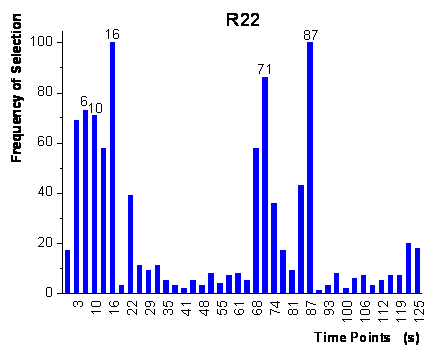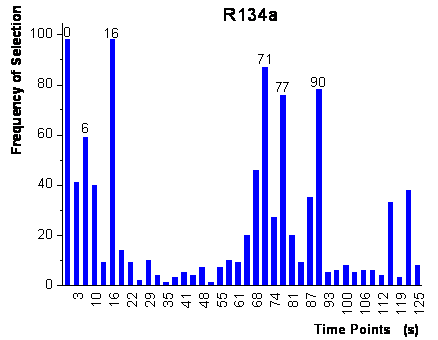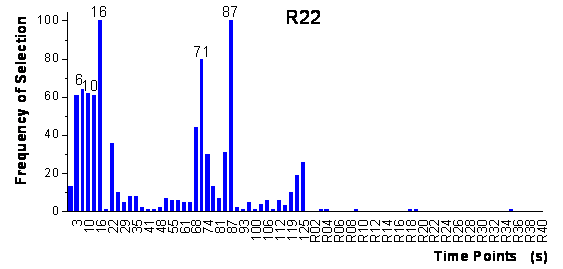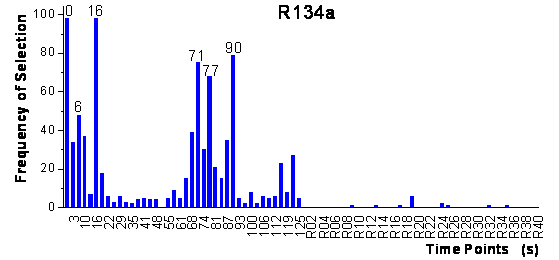Home News About Me Ph. D. Thesis Abstract Table of Contents 1. Introduction 2. Theory  Fundamentals of the Multivariate Data Analysis 3. Theory  Quantification of the Refrigerants R22 and R134a: Part I 4. Experiments, Setups and Data Sets 5. Results  Kinetic Measurements 6. Results  Multivariate Calibrations 7. Results  Genetic Algorithm Framework 8. Results  Growing Neural Network Framework 8.1. Modifications of the Growing Neural Network Algorithm 8.2. Application of the Growing Neural Networks 8.3. Growing Neural Network Algorithm Frameworks 8.4. Applications of the Growing Neural Network Frameworks 8.4.1. Parallel Framework 8.4.2. Loop-based Framework 8.5. Conclusions and Comparison of the Different Methods 9. Results  All Data Sets 10. Results  Various Aspects of the Frameworks and Measurements 11. Summary and Outlook 12. References 13. Acknowledgements Publications Research Tutorials Downloads and Links Contact Search Site Map Print this Page## 8.4.   Applications of the Growing Neural Network Frameworks

### 8.4.1.   Parallel Framework

For the parallel approach, 100 runs of the growing neural network algorithm were performed simultaneously for each analyte. The ranking of the variables after the first step of the frameworks is shown in figure 55. In contrast to the genetic algorithm framework, the variables are ranked separately for each analyte, as for each analyte separate networks were grown. The final topology resulted in fully connected networks for R22 with 5 input neurons and 6 hidden neurons and for R134a with 6 input neurons and 7 hidden neurons. The input variables, which were used by these networks, are labeled in figure 55. These networks were subsequently trained with the complete calibration data set and then used for the prediction of the validation data whereby the errors are shown in table 4. The predictions of the validation data not used during the network building process are the best out of all methods used up to now with 2.04% for R22 and 2.61% for R134a. The small size of the networks with only 43 respectively 57 adjustable parameters is rewarded by an excellent generalization ability. The true-predicted plots look pretty much like the true-predicted plots of the genetic algorithm framework (figure 47) with low standard deviations and no bias present and are not shown here. For both analytes, all variables used by the networks were recorded within the first 16 seconds of exposure to analyte and within the first 30 seconds after the end of exposure to analyte (60 seconds to 90 seconds). The selected time points are located within the same time intervals as the time points selected by the genetic algorithm framework (except of the time-point 125 s). Yet, the parallel growing neural network framework achieves better predictions using fewer variables and smaller networks. Additionally, the variable selection of the parallel framework suggests to reduce the exposure time to 20 seconds and to record the signal of the sensor for 90 seconds. The reduced time of exposure to analyte would also reduce the time needed for the recovery of the sensor signal resulting in a significantly shorter repetition times.figure 55:  Ranking of the time-points represented as frequency of being present in the grown neural networks of the first step of the parallel framework.

The same test for chance correlation and reproducibility was performed as for the genetic algorithm framework before. The parallel framework was used for the increased data set with 40 additional autoscaled random variables the same way as described for the original data set. In the ranking of the variables after the first step, no random variable was found in the top 34 variables for R22 and in the top 25 variables for R134a. This means that the growing neural network algorithm and especially the parallel framework are very robust to selecting randomly correlated variables. In figure 56, it is shown that for R22 the first 8 variables and for R134a the first 7 variables in the ranking were exactly the same as in the previous run of the parallel algorithm resulting in exactly the same neural network topologies and predictions. Thus, the parallel framework shows a high reproducibility not sensitive to the partitioning of the calibration data set.figure 56:  Ranking of the time-points and of the random variables after the first step of the parallel framework for the increased data set.

 Page 110 © Frank Dieterle, 03.03.2019